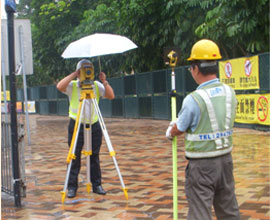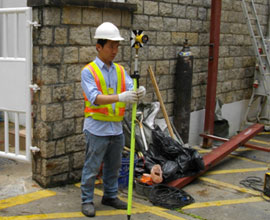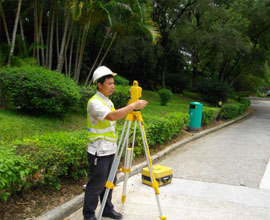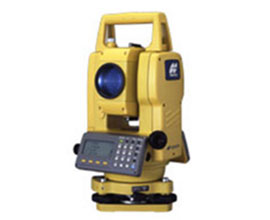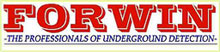FORWIN Site Investigation Engineering Ltd.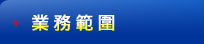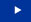地下管線探測地下帶水設備勘察工作管道滲漏探測地型測量快速紅外線檢測結構測試探測工程内容    地型測量

Condition Survey & Topographical Survey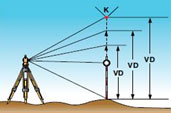Remote elevation measurement(REM)
This feature measures the elevation of a point where a prism can not be placed directly.The mesurement is extended along the plumb line while the elevation is continuously displayde.

Missing line measur measurement(MLM)
Multiple lines can be drawnbetween;
‧the first point and the last point;
‧ the last 2 points.
Horizontal distance,difference in height and slope distance is calculated.Coordinate file data and manual input data are available for further use.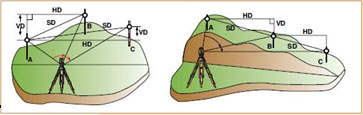Plane offset measurement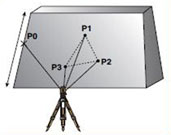Coordinates are calculated for points where direct measurements to a prism can not be taken,for example point-measurements on a wall or plane.Three random points（P1,P2,P3）on the plane will be measured first to determine the measured plane and their angles and distances are temporarily stored,Then sight the unknow point on the plane and the instument calculates and displays coordinates and distance values of desired point

Z coordinate of occupied point(benchmark elevation)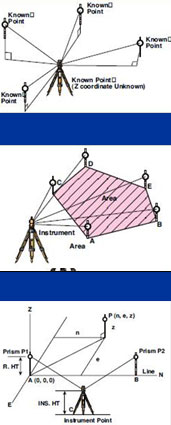The Z-coordinate and direction angle of the instrument is calculated and reset by measuring Z-coordinates of known pointa(Max.10 points).

Area cakulation
Area is calculated using measured data or file data (Coordinate data).

Point-to-line measurement
Create a new coordinate by measuring to two points.The first point becomes the origin and the secound point becomes the Naxis direction.